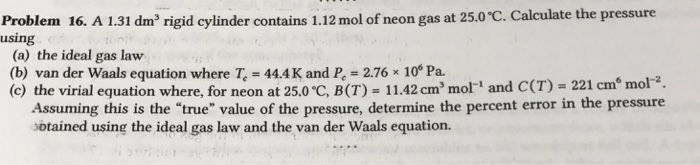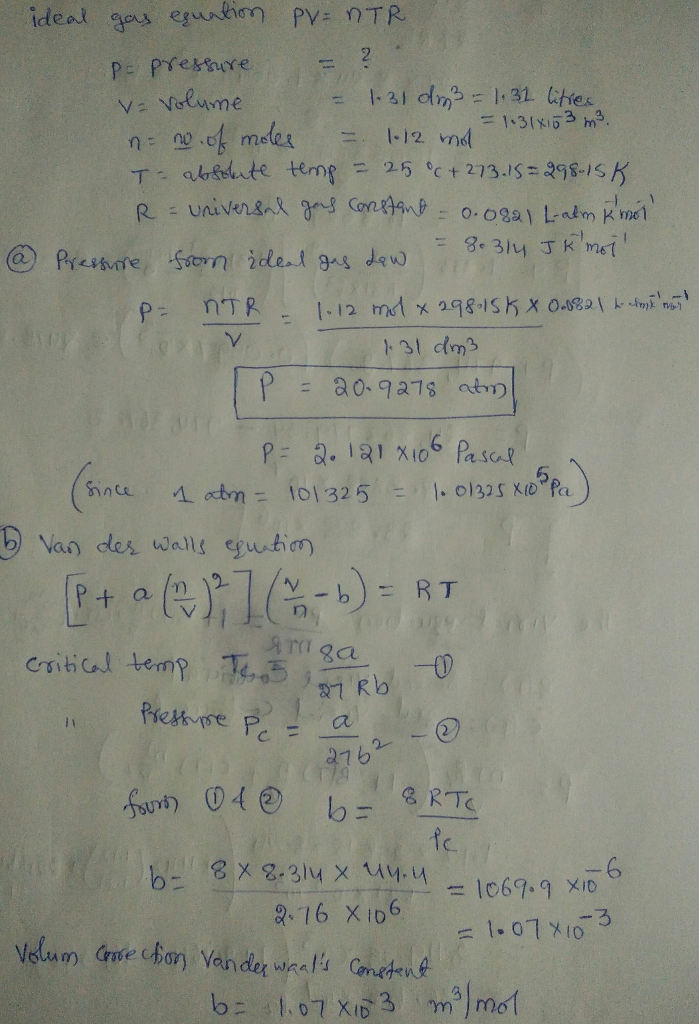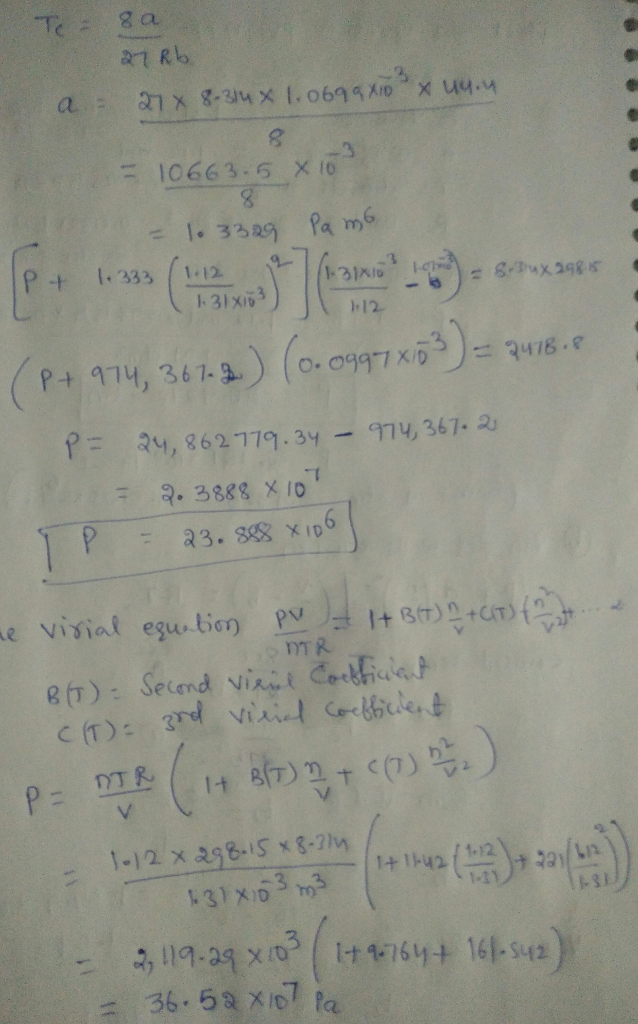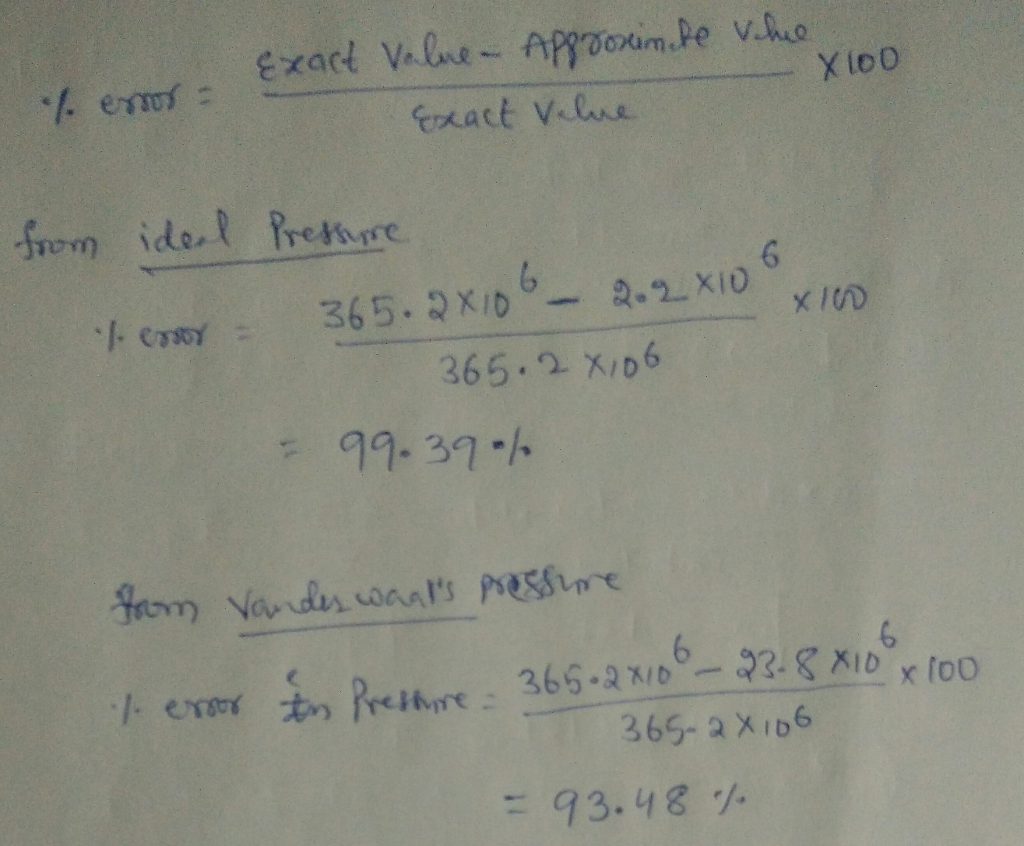# Question & Answer: A 131 dm^3 rigid cylinder contains 1.12 mol of neon gas at 25.0 degree C. Calculate the…..A 131 dm^3 rigid cylinder contains 1.12 mol of neon gas at 25.0 degree C. Calculate the pressure using (a) the ideal gas law (b) van der Waals equation where T_c = 44.4K and P_c = 2.76 times 10^6 Pa. (c) the virial equation where, for neon at 25.0 degree C, B (T) = 11.42 cm^3, mol^-1 and C(T) = 221 cm^6 mol^-2. Assuming this is the “true” value of the pressure, determine the percent error in the pressure obtained using the ideal gas law and the van der Waals equation.